Courses

# Test: Motion - 4

## 25 Questions MCQ Test General Science(Prelims) by IRS Divey Sethi | Test: Motion - 4

Description
This mock test of Test: Motion - 4 for UPSC helps you for every UPSC entrance exam. This contains 25 Multiple Choice Questions for UPSC Test: Motion - 4 (mcq) to study with solutions a complete question bank. The solved questions answers in this Test: Motion - 4 quiz give you a good mix of easy questions and tough questions. UPSC students definitely take this Test: Motion - 4 exercise for a better result in the exam. You can find other Test: Motion - 4 extra questions, long questions & short questions for UPSC on EduRev as well by searching above.
QUESTION: 1

### A body moves in a uniform circular motion

Solution: Accelerating objects are objects which are changing their velocity - either the speed (i.e., magnitude of the velocity vector) or the direction. An object undergoing uniform circular motion is moving with a constant speed. Nonetheless, it is accelerating due to its change in direction.
QUESTION: 2

Solution:
QUESTION: 3

### Speed of a body in particular direction can be called

Solution: Distance covered by a body in per unit time is called Speed & Distance covered by a body in a given direction is known as Velocity ....... It can also be written as speed of a body in a particular direction is called Velocity .
QUESTION: 4

Statement A: Uniform circular motion is a case of accelerated motion
Statement B: In third equation of motion we do not have the time.

Solution:
QUESTION: 5

Which of the following is correct about uniform circular motion?
A. Direction of motion is continuously changed
B. Direction of motion is not changed
C. Speed and direction both remain constant
D. Speed is constant, but direction is changing

Solution:

Uniform circular motion is accelerated because the velocity changes due to continuous change in the direction of motion. So, even when the body moves ta a constant speed, its velocity is not constant. Thus, for a body moving in a circular path at a constant speed, its velocity changes continuously. The change in velocity gives rise to an acceleration in the moving body. Therefore circular motion is an acceleration motion even when the speed remains constant.

QUESTION: 6

Which of the quantities – speed, time, velocity and acceleration have the same SI unit?

Solution:
QUESTION: 7

Find the correct statement

Solution:
QUESTION: 8

A body is said to be in rest when

Solution:  If philosophically speaking one could be physically at rest but mentally in motion for example when one is taking a nap, except one is dead, one's internal system is constantly in motion as breathing occurs, dreams, digestion etc. Rest is a state in physics that refers to an object being stationary relative to a particular frame of reference or another object; when the position of a body with respect to its surroundings does not change with time it is said to be "at rest".
QUESTION: 9

A bus moves from stop ‘A to stop ‘B’ with a speed of 40 km/hr and then from stop ‘B’ to stop ‘A with a speed of 50 km/hr. Its average speed is

Solution:

Let the distance between A and B be D and time taken to cover the distance be T1 and T2.

Speed = Distance/Time

40 = (D/T1) and 50 = (D/T2)

T1 = (D/40)

also, T2 = (D/50)

Total time (T) = T1 + T2

⇒ (D/40) + (D/50) = (9D/200)

Total Distance = (D + D) = 2D

Average speed = Total Distance/Total Time Taken

= (2D/(9D/200)) = 400/9 = 44.4 km/hr

QUESTION: 10

Find the incorrect statement

Solution:
QUESTION: 11

Match the following with correct response.Solution:
QUESTION: 12

Match the following with correct response.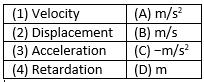Solution:
QUESTION: 13

Match the following with correct response.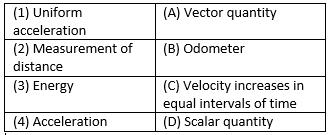Solution:
QUESTION: 14

The area under the velocity – time graph gives the

Solution:
QUESTION: 15

Which of the following is a false statement

Solution: A body can have a constant speed but a changing velocity because the direction can change while the speed is constant. (A car goes 50 mph around a roundabout). However, a body can not have a constant velocity with a changing speed.
QUESTION: 16

Is the following graph possible ?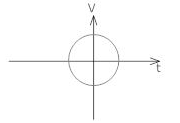Solution:
QUESTION: 17

Which of the following situations are not possible?

Solution:
QUESTION: 18

If we get a curve line in displacement – time graph then the velocity is

Solution:
QUESTION: 19

Match the following with correct response.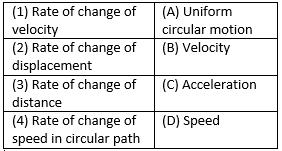Solution:
QUESTION: 20

Match the following with correct response.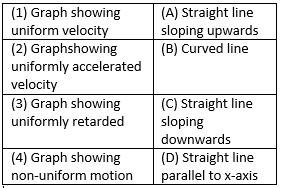Solution:
QUESTION: 21

Which of the following statement is correct?
A. When a body starts from rest, u = 0
B. When a body comes to rest, V = 0
C. For a body under free fall, a = −9.8m/s2
D. For uniform velocity, a = 0

Solution:
QUESTION: 22

The slope of distance – time graph gives instantaneous.

Solution: The gradient of a distance-time graph represents the speed of an object. The velocity of an object is its speed in a particular direction. The slope on a velocity-time graph represents the acceleration of an object. The distance travelled is equal to the area under a velocity-time graph.
QUESTION: 23

Distance is a __________ quantity

Solution:

Distance is a scalar quantity that refers to "how much ground an object has covered" during its motion.

QUESTION: 24

If a body doesn't rotates then its motion is

Solution: The correct answer is B because this applies in the case of inertia. if you are travelling on a vehicle you are not doing and movement but the vehicle is moving then at that time you are at rest as well as at motion.
QUESTION: 25

A body is moving with a velocity of 10m/s. If the motion is uniform, what will be the velocity after 10 second?

Solution:

As the body is moving in uniform motion, so the acceleration will be zero.
as acceleration is zero, the change in velocity is also zero.it means velocity will be the same in whole motion.